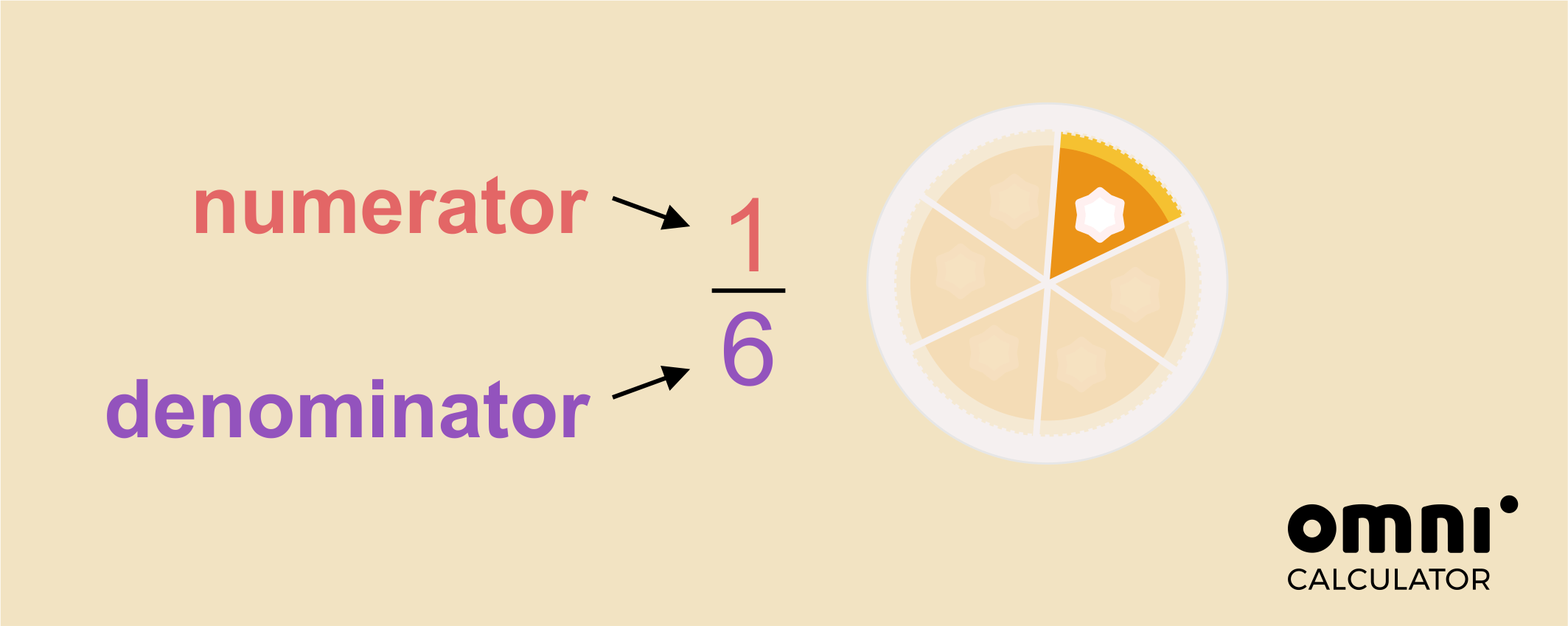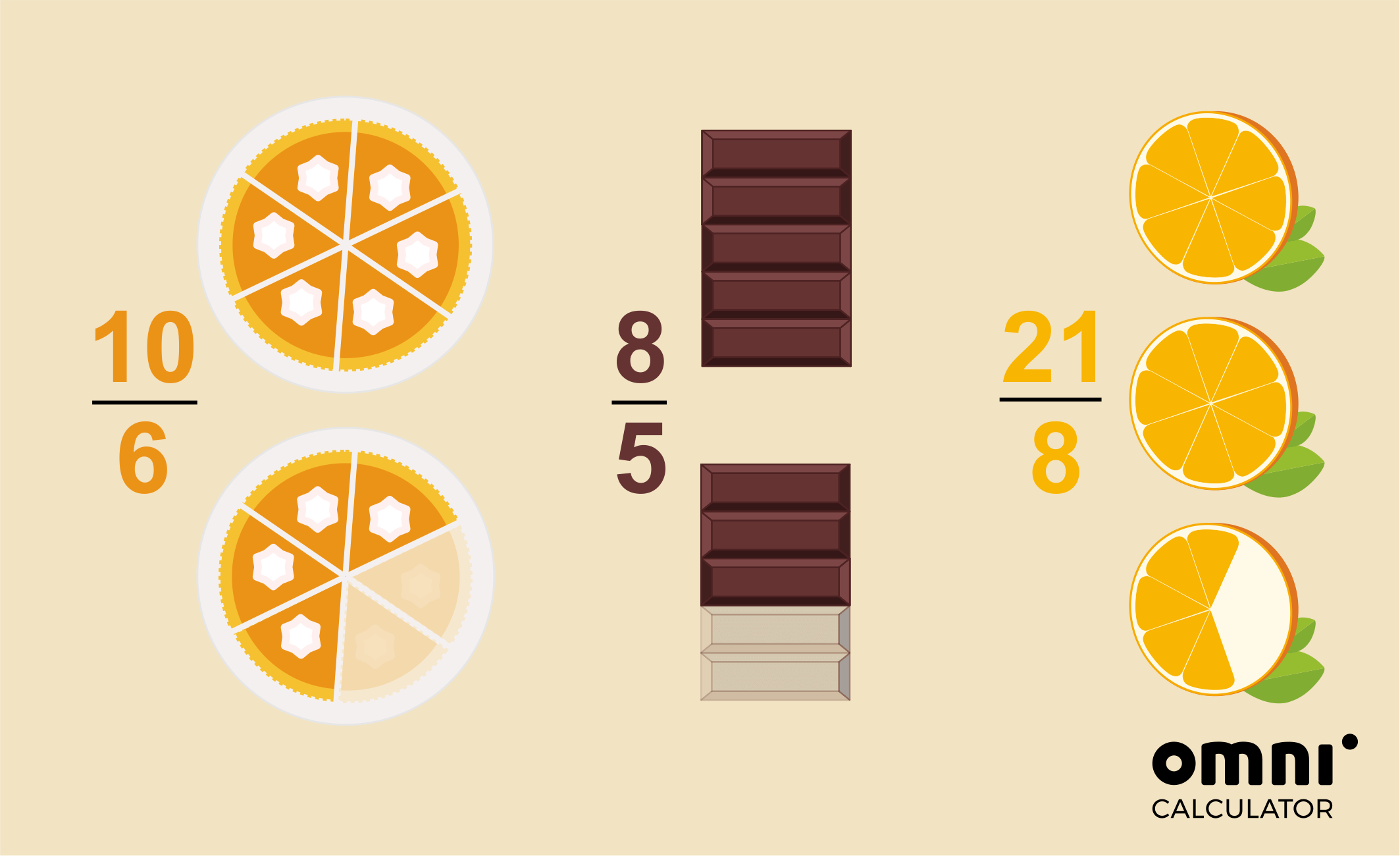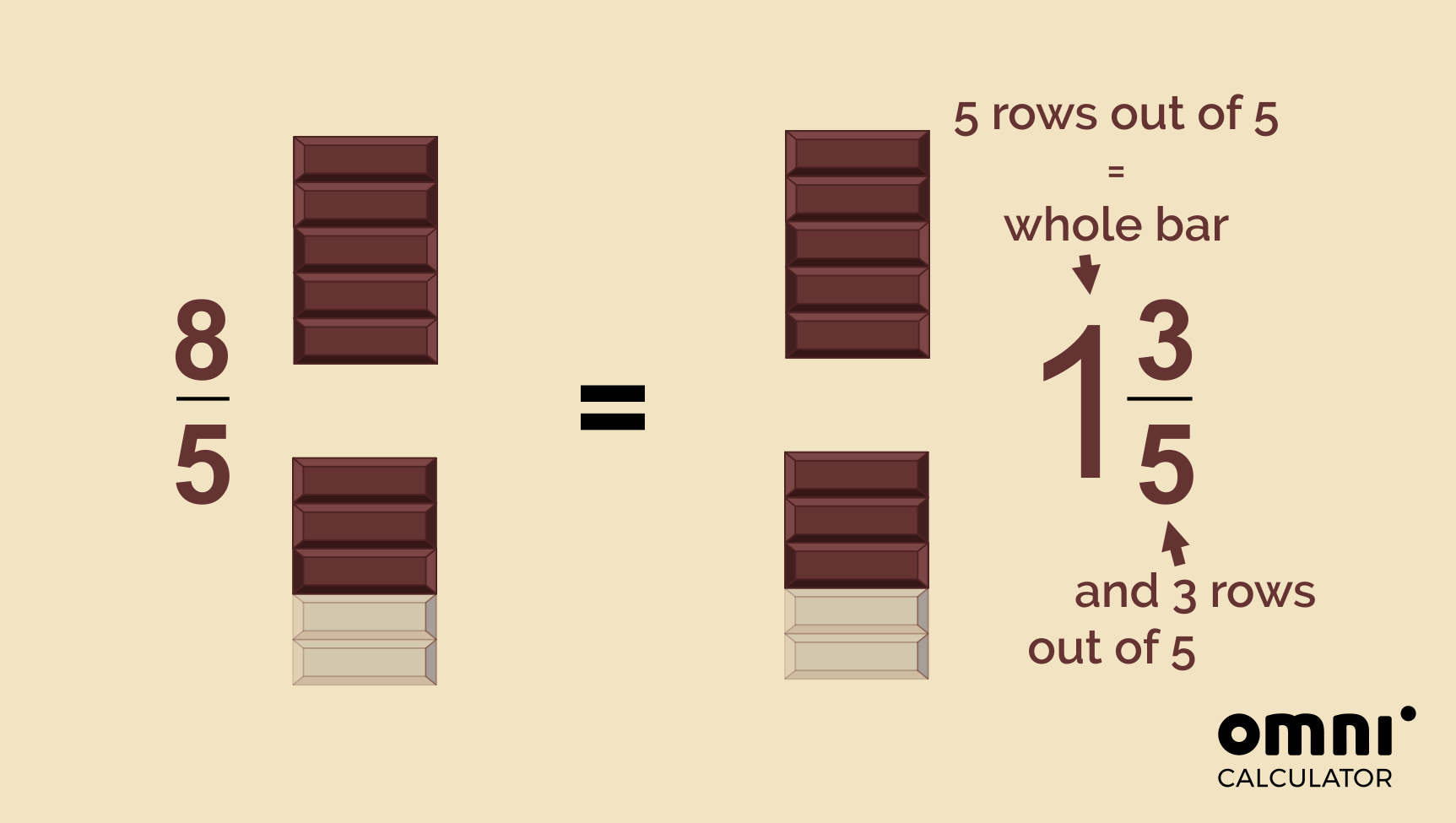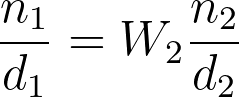# Improper Fraction to Mixed Number Calculator

Created by Hanna Pamuła, PhD candidate
Reviewed by Dominik Czernia, PhD candidate and Jack Bowater
Last updated: Aug 02, 2022

Welcome to the improper fraction to mixed number calculator - a tool which lets you convert an improper fraction to a mixed number in the blink of an eye. In the short article below, you'll learn the basics - such as what an improper fraction is. Also, we will show you step-by-step instructions on how to turn an improper fraction into a mixed number.

Since you're already here, peeking 👀 at this improper fraction to mixed number calculator, we suspect that you may be interested in other fraction topics:

If you don't want to go into too much detail, we've built the comprehensive fractions calculator that can deal with seven basic fraction operations.

## What is an improper fraction? Improper fraction definition

To talk about improper fraction definition, we need to start with a small bit of revision - what is a fraction, in general?

💡 A fraction tells us how many equal parts of a whole thing we have. We write it as a ratio of whole numbers, that are separated by line or a slash.

We call the top number the numerator and the bottom one the denominator.So in the above example, we have 1 part out of 6 - 1/6 read as one-sixth.

Now we can come back to our key question: what is an improper fraction? According to the improper fraction definition:

💡 An improper fraction is the fraction when the numerator is larger than (or equal to) the denominator.

As the top number is equal or greater than the bottom number, we sometimes call the improper fraction a top-heavy fraction. The examples of the improper fractions are:

• 🍰 10 slices of a cake, when each cake was cut to 6 slices,

• 🍫 8 rows of the chocolate bar, if the whole chocolate bar has 5 rows,

• 🍊 21 parts of orange, if we cut each orange to 8 equal pieces.## How to turn an improper fraction into a mixed number?

So, what does converting improper fractions to mixed numbers mean? All in all, a mixed fraction is just another way of expressing an improper fraction. But how does it work?

Look at the previous image again. In each of these examples, we have more than one whole thing, right? So, for example, instead of saying we have 8 rows of chocolate (and each bar has 5 rows), we could say - hey, I've one whole chocolate bar and an additional 3 parts out of 5. And that's exactly how converting improper fractions to mixed numbers works!Let's go through our example step-by-step to see how to turn an improper fraction into a mixed number:

1. Divide the numerator by the denominator (the top number by the bottom number).

If you divide 8 by 5, you get a quotient (whole number) and a remainder:

8/5 = 1 remainder 3 = 1 R 3

2. Find the whole part of the mixed number - take the whole number from the division

1

3. Find the fractional part of the mixed number - the remainder from the division is the new numerator, while the denominator stays the same:

3/5

4. To get the mixed number, put the numbers together:

1 3/5

And that's it, 8/5 = 1 3/5

Sometimes you should also simplify the mixed fraction, but that's not the case here.

## Improper fractions to mixed numbers calculator - an example

So, let's quickly check how converting improper fractions to mixed numbers works in this tool. Let's say you have fraction 81/17 and you'd like to find it's equivalent as a mixed number:

1. Enter the numerator of the improper fraction. It's 81.
2. Input the denominator. 17 in our case.
3. And that's it - the improper fraction to mixed numbers calculator shows the result:
Improper
fraction
Mixed
number
81/17 4 13/17

Could it be more straightforward? 🙂

Hanna Pamuła, PhD candidateImproper fraction
Numerator (n₁)
Denominator (d₁)
Step by step solution?
No
People also viewed…

### Area of obtuse triangle

The area of an obtuse triangle calculator contains the formulas to count the area of that type of triangle and does it automatically for you.

### Coffee kick

A long night of studying? Or maybe you're on a deadline? The coffee kick calculator will tell you when and how much caffeine you need to stay alert after not sleeping enough 😀☕ Check out the graph below!

### Lost socks

Socks Loss Index estimates the chance of losing a sock in the laundry.

### Volume of a hemisphere

Our volume of a hemisphere calculator is designed to answer the questions: How can you find the volume of a hemisphere? What is the volume of a hemisphere formula?